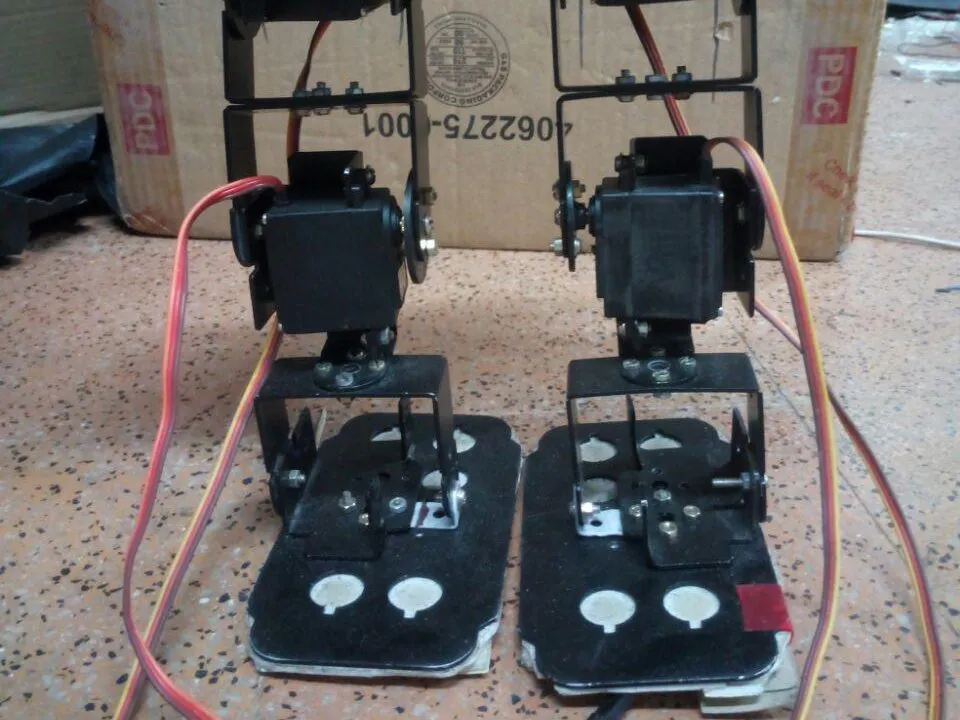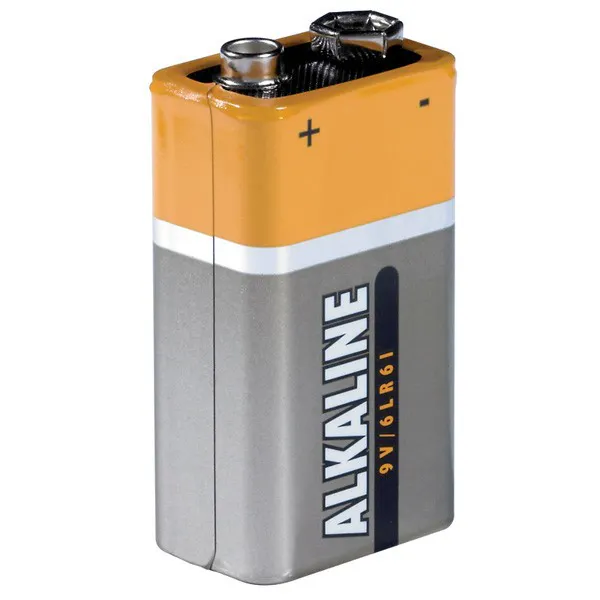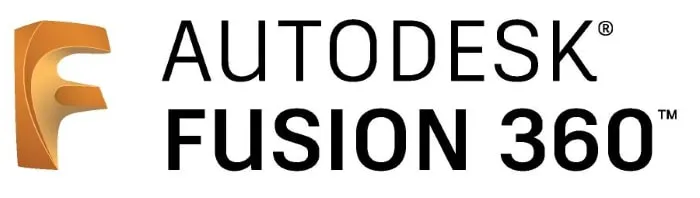# Blynk Controlled BIPED

A biped controlled by using Blynk app.## Things used in this project

### Hardware components

 Servos (Tower Pro MG996R)
×6Jumper wires (generic)
×149V battery (generic)
×1
 Servo Shield
×1
 Double Side Tape
×1

### Software apps and online servicesArduino IDEAutodesk Fusion 360

### Hand tools and fabrication machines

 Driller

## Custom parts and enclosures

The Design which we implemented.

## Schematics

### Servo Connection

Servo are Connected in this manner, based on which servo is assigned which pin on the shield. It is clearly mentioned on the program.

## Code

### Arduino Code

C/C++
This code is the final that gets uploaded to the arduino.
```#include <Servo.h>
Servo a1,a2,b1,b2,c1;
int x;
String z;
char y;
int d=90;
int h=90;
int s=0,i=0,j=90,t=90;
int k=0,a=0,l=0,p=0,f=1,q=0,o=0;
long duration, distance;

void setup()
{
Serial.begin(9600);
a1.attach(11);
a2.attach(10);
b1.attach(9);
b2.attach(5);
c1.attach(3);
a1.write(90);
a2.write(90);
b1.write(90);
b2.write(90);
c1.write(95);
pinMode(12, OUTPUT);
pinMode(8, INPUT);
pinMode(2,OUTPUT);
digitalWrite(2,HIGH);
}

void loop()
{
if(Serial.available()>0)
{
y= z[z.length()-1];
}
digitalWrite(12, LOW);
delayMicroseconds(2);

digitalWrite(12, HIGH);
delayMicroseconds(10);

digitalWrite(12, LOW);
duration = pulseIn(8, HIGH);
distance = duration/58.2;
if(distance>=25||distance==0)
{
y=y;
}
else
{
y='s';
}
if(y=='f')
{
if(s==0)
{
// delay(5000);
c1.write(105);
delay(600);
while(k==0)
{
c1.write(105);
if(a==0)
{
k=1;
}
}
c1.write(95);
delay(100);
k=0;
}
for(i=90;i<=105;i+=1)
{
a1.write(i);
delay(30);
a2.write(j);
j=j-1;
delay(30);
}
delay(100);
for(i=90;i<=105;i+=1)
{
b1.write(i);
delay(30);
b2.write(t);
t=t-1;
delay(30);
}
delay(100);
c1.write(83);
delay(800);
while(k==0)
{
c1.write(83);
if(a==0)
{
k=1;
}
}
c1.write(95);
delay(200);
k=0;

c1.write(83);
delay(800);
while(k==0)
{
c1.write(83);
if(a==0)
{
k=1;
}
}
c1.write(95);
delay(100);
k=0;
for(i=x;i>=90;i-=1)
{
a1.write(i);
delay(30);
a2.write(x);
x=x+1;
delay(30);
}
delay(100);
for(i=x;i>=90;i-=1)
{
b1.write(i);
delay(30);
b2.write(x);
x=x+1;
delay(30);

}
delay(100);
for(i=90;i>=75;i-=1)
{
b1.write(i);
delay(30);
b2.write(d);
d=d+1;
delay(30);
}
delay(100);
for(i=90;i>=75;i-=1)
{
a1.write(i);
delay(30);
a2.write(h);
h=h+1;
delay(30);
}
c1.write(105);
delay(400);
while(k==0)
{
c1.write(105);
if(a==0)
{
k=1;
}
}
k=0;
c1.write(95);
delay(200);
c1.write(105);
delay(400);
while(k==0)
{
c1.write(105);
if(a==0)
{
k=1;
}
}
k=0;
c1.write(95);
delay(100);
for(i=x;i<=90;i++)
{
b1.write(i);
delay(30);
b2.write(x);
x=x-1;
delay(30);
}
delay(100);
for(i=x;i<=90;i++)
{
a1.write(i);
delay(30);
a2.write(x);
x=x-1;
delay(30);
}
delay(100);
/*c1.write(83);
delay(400);
while(k==0)
{
c1.write(83);
if(a==0)
{
k=1;
}
}
c1.write(95);
delay(100);*/
k=0;
d=90;
h=90;
t=90;
j=90;
s=1;
l=1;
f=0;
q=1;
}
//delay(50);
else if(y=='s')
{
if(l==1)
{
delay(100);
c1.write(83);
delay(800);
while(k==0)
{
c1.write(83);
if(a==0)
{
k=1;
}
}
c1.write(95);
delay(100);
k=0;
}
f=1;
p=0;
s=0;
l=0;
o=0;
q=0;
}
else if(y=='k')
{
if(f==1)
{
k=0;
c1.write(105);
delay(400);
while(k==0)
{
c1.write(105);
if(a==0)
{
k=1;
}
}
k=0;
c1.write(95);
delay(200);
}
f=0;

if(p==0)
{
/*c1.write(83);
delay(800);
while(k==0)
{
c1.write(83);
if(a==0)
{
k=1;
}
}
c1.write(95);
delay(100);
k=0;*/
for(i=x;i<=145;i++)
{
a1.write(i);
delay(30);
a2.write(x);
x=x-1;
delay(30);
}
delay(100);
//
for(i=x;i>=30;i--)
{
a2.write(i);
delay(30);
}

delay(100);

for(i=x;i>=100;i--)
{
a1.write(i);
delay(30);
}
delay(100);
for(i=x;i<=105;i++)
{
b1.write(i);
delay(60);
b2.write(x);
x=x-1;
delay(60);
}
delay(100);
for(i=x;i>=55;i--)
{
a1.write(i);
delay(30);
}
delay(100);
for(i=x;i<=100;i++)
{
a1.write(i);
delay(30);
}
delay(100);
for(i=x;i>=90;i--)
{
b1.write(i);
delay(60);
b2.write(x);
x=x+1;
delay(60);
}
delay(200);
for(i=x;i<=145;i++)
{
a1.write(i);
delay(30);
}

delay(100);
for(i=x;i<=35;i++)
{
a2.write(i);
delay(30);
}
delay(100);
for(i=x;i>=90;i-=1)
{
a1.write(i);
delay(30);
a2.write(x);
x=x+1;
delay(30);
}
delay(100);
//
c1.write(83);
delay(800);
while(k==0)
{
c1.write(83);
if(a==0)
{
k=1;
}
}
c1.write(95);
delay(100);
k=0;
p=1;
l=0;
delay(50);
}
}
else if(y=='l')
{
if(q==1)
{
c1.write(83);
delay(800);
while(k==0)
{
c1.write(83);
if(a==0)
{
k=1;
}
}
c1.write(95);
delay(100);
k=0;
}
q=0;
delay(100);
if(o==0)
{
for(i=x;i<=105;i+=1)
{
b1.write(i);
delay(30);
b2.write(x);
x=x-1;
delay(30);
}
delay(3000);
for(i=x;i>=90;i--)
{
b1.write(i);
delay(30);
b2.write(x);
delay(30);
x=x+1;
}
delay(100);
}
o=1;
}
}
```

## Credits

### Akash Ravichandran

15 projects • 87 followers
Developer and a Learner